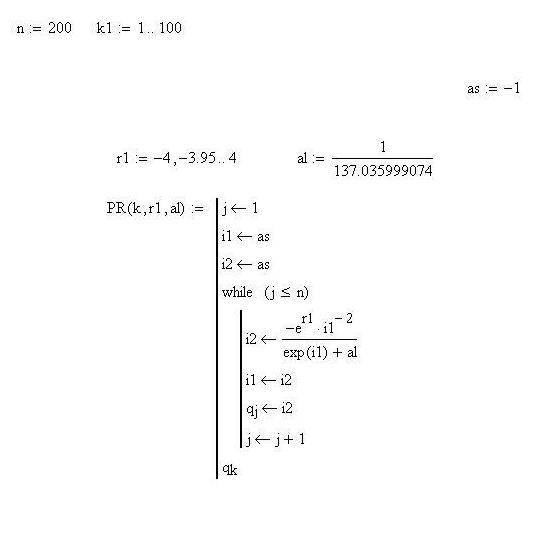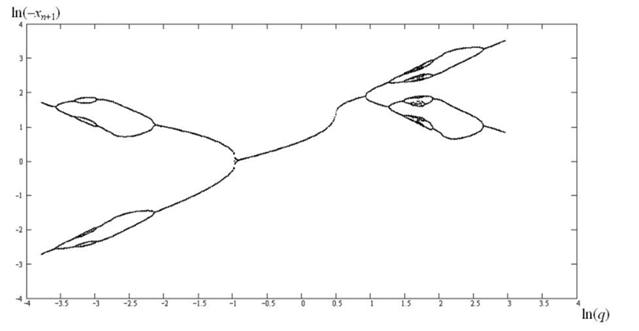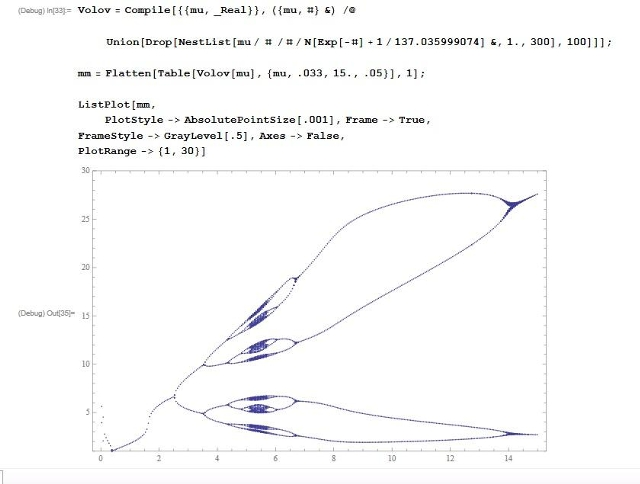Scale Theory / Масштабная теорияReferences / Список литературыArchive / АрхивДинамика Ферхюльста-Рикера-Планка и ее связь с постоянной тонкой структурыThe Verhulst-Ricker-Planck dynamic and its relation to the fine structure constantNuclear physics, A.P. Trounev (Canada, Toronto) The VRP-2 dynamics, with the parameter alpha value approaching the value equal to the value of the dimensionless ﬁne-structure constant, features maximum variety with regard to the number of bifurcations with the minimum degree of chaosticity. D. B. Volov Specific behavior of one chaotic dynamics near the fine-structure constant http://arxiv.org/abs/1205.6091http://chaosandcorrelation.org/Chaos/DV_1_5_2012.pdfVolov_D__VTP_5_2011.pdfhttp://www.sciteclibrary.ru/rus/catalog/pages/11612.htmlThis files contains an articles describing the Verhulst-Ricker-Planck dynamic and its relation to the fine structure constant.The MathCAD's text program for the bifurcation diagram "four rats" (D.B.Volov, Russia, Samara).Diagram "four rats"  Source code in MATLAB to reproduce the bifurcation diagram "four rats" (A.P.Trounev, Toronto, Canada)(smile must be replaced by a colon) x(i+1)=-L(k)/(x(i)^2(exp(x(i))+alpha))L=zeros(1,500);y=zeros(1,128);ly=zeros(1,128);alpha=1/137.035999074;dL=(3.7+exp(1.1989))/500;for k=1:length(L)    for i=1:length(y)        if (k==1)            L(k)=-3.8;            y(k,=1;            ly(k,=log(y(k,);        else            if (i==1)                L(k)=L(k-1)+dL;                V=y(k-1,128)*y(k-1,128)*(exp(-y(k-1,128))+alpha);                y(k,i)=exp(L(k))/V;               ly(k,i)=log(y(k,i));            else                V1=y(k,i-1)*y(k,i-1)*(exp(-y(k,i-1))+alpha);                y(k,i)=exp(L(k))/V1;                 ly(k,i)=log(y(k,i));            end        end    endendfor i=1:128    plot(L,ly(:,i),'.k')    hold on    title('a=1/137');    xlabel('lnK');ylabel('lnx');endThe result: http://chaosandcorrelation.org/AS/4rat.gif The bifurcation diagram "four rats" one-dimensional dynamic. Code for the Wolfram Mathematica 8 & result (A.P.Trounev, Toronto, Canada):